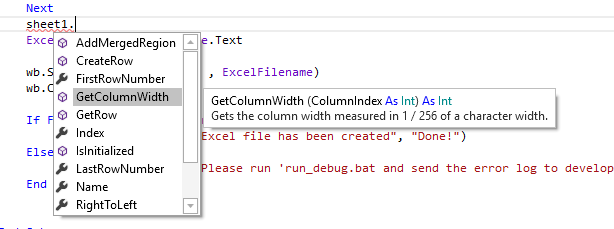# B4J TutorialjPOI Library - Automatic column width.

I was trying to get to grips with setting the column width in my Excel table. According to the help I tried:

number of characters x 256, but all my columns dissappeared!Setting a value of 2560 gives a width in Excel of 9.29 or 70 Pixels, enough for 9 characters. 2560/9.29=275 which I rounded to 285

I finally got this code which will adjust according to the length of the data.
It may not be pretty but it works!

Hope it helps someone.

B4X:
``````'Put the values from Data into a new Excel worksheet
Sub SendDataToExcel
Dim wb As PoiWorkbook
wb.InitializeNew(True) 'create new xlsx workbook
Dim sheet1 As PoiSheet = wb.AddSheet("Flug Buch", 0)
Dim Columnwidth(23),Cw As Int

For i=0 To CurrentLine
Dim row As PoiRow = sheet1.CreateRow(i)

For j=0 To 20
row.CreateCellString(j,data(i,j))
Cw=(data(i,j).Length+1)*285                        '<= Calculated column width based on length of string
If Cw>Columnwidth(j) Then Columnwidth(j)=Cw        'store new column width if it is higher
sheet1.SetColumnWidth(j,Columnwidth(j))            'Set new width
Next
Next

ExcelFilename=txtExcelFile.Text

wb.Save(lblDirectory.Text , ExcelFilename)
wb.Close

If File.Exists(lblDirectory.Text , ExcelFilename) Then
fx.Msgbox(MainForm, "Excel file has been created", "Done!")
Else
fx.Msgbox(MainForm, "Please run 'run_debug.bat and send the error log to developer'", "ERROR")
End If

End Sub``````

•Mashiane and Erel